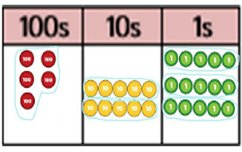# Beckstone Primary School

Bridging communities | Promoting learning and excellence | Shaping the future.

Eadie Street, Harrington, Workington, Cumbria CA14 5PX
01946 830423 | wb@beckstone.cumbria.sch.uk |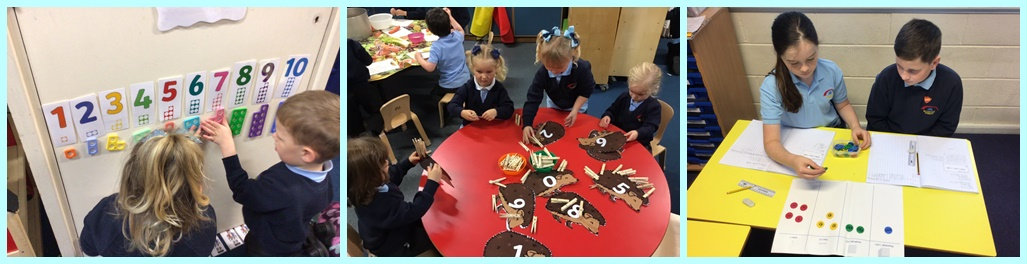The following calculation policy has been devised to meet requirements of the National Curriculum 2014 for the teaching and learning of mathematics, and is also designed to give pupils a consistent and smooth progression of learning in calculations across the school. Please note that early learning in number and calculation in the Foundation Stage follows the Development Matters EYFS document and this calculation policy is designed to build on progressively from that content and methods established in the Early Years Foundation Stage.

##### How to use the policy

This mathematics policy is a guide for all staff and has been adapted from the work by the NCETM and the White Rose Maths Hub. It is purposely set out as a progression of mathematical skills and not year group stages to encourage a flexible approach to teaching and learning. It is expected that teachers will use their professional judgement as to when consolidation of existing skills is required or if to move onto the next concept. However the focus must always remain on breadth and depth rather than accelerating through the concepts. Children should not be extended with new learning before they are ready, they should deepen their conceptual understanding by tackling challenging and varied problems.

##### Providing a context for calculation:

It is important that any type of calculation is given a real life context or problem solving approach to help build children’s understanding of the purpose of calculation and to help them recognise when to use certain operations and methods when faced with problems. This must be a priority within calculation lessons.

##### Choosing a calculation method:

At the centre of the mastery approach to the teaching of mathematics it is the belief that all children have the potential to succeed. They should access the same curriculum content and, rather than being extended with new learning, they should deepen their conceptual understanding by tackling challenging and varied problems. Similarly, with calculation strategies, children must not be simply rote learn procedures but demonstrate their understanding of these procedures through the use of concrete materials and pictorial representations. This policy outlines the different calculation strategies that should be taught and used in Year 1 to Year 6 in line with the requirements of the 2014 National Curriculum.

Teachers can use any teaching resources that they wish to use and the policy does not recommend one set of resources over another, rather that, a variety of resources are used. For each of the four rules of number, different strategies are laid out together with examples of what concrete materials can be used and how, along with suggested pictorial representations. The principal of the concrete-pictorial-abstract (CPA) approach is for the children to have a true understanding of a mathematical concept, we aim for the children to be secure using abstract methods by the end of the year group’s scheme of work but also confident to use pictorial methods when they need to.

Please click on a tab below.

##### EYFS/Year 1

Combining two parts to make a whole: part whole model

Starting at the bigger number and counting on using cubes.

Regrouping to make 10 using ten frame.

##### Year 2

Use of base 10 to combine two numbers.

##### Year 3

Column method-regrouping.

Using place value counters (up to 3 digits).

##### Year 4

Column method-regrouping.

Using place value counters (up to 4 digits).

##### Year 5

Column method-regrouping.

Use of place value counters for adding decimals.

##### Year 6

Column method-regrouping.

Abstract methods.

Place value counters to be used for adding decimal numbers.

##### Concrete

Combining two parts to make a whole (use other resources too e.g. eggs, shells, teddy bears, cars).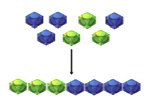##### Pictorial

Children to represent the cubes using dots or crosses. They could put each part on a part whole model too.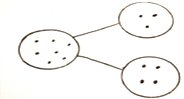##### Abstract

4 + 3 = 7
Four is a part, 3 is a part and the whole is seven.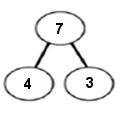Counting on using number lines using cubes or Numicon.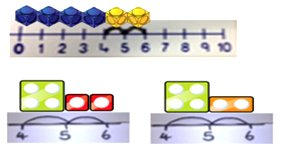A bar model which encourages the children to count on, rather than count all.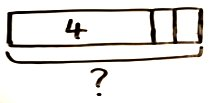The abstract number line:
What is 2 more than 4?
What is the sum of 2 and 4?
4 + 2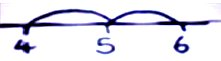Regrouping to make 10; using ten frames and counters/cubes or using Numicon.
6 + 5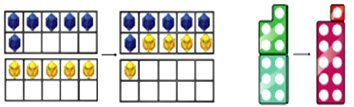Children to draw the ten frame and counters/cubes.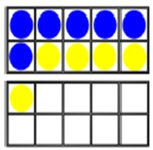Children to develop and understanding of equality e.g.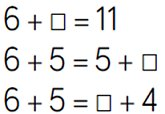TO + O using base 10 Continue to develop understanding of partitioning and place value
41 + 8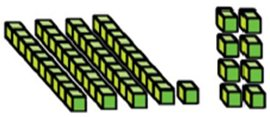Children to represent the base 10 e.g. lines for tens and dot/crosses for ones.TO + TO using base 10. continue to develop understanding of partitioning and place value
36 + 25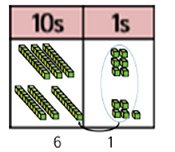Children to represent the base 10 in a place value chart.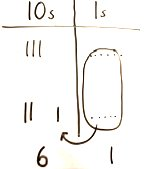Looking for ways to make 10.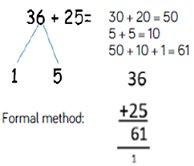Use of place value counters to add HTO + TO, HTO + HTO etc. When there are 10 ones in the 1s column - we exchange for 1 ten, when there are 10 tens in the 10s column - we exchange for 1 hundred.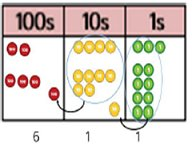Children to represent the counters in a place value chart, circling when they make an exchange.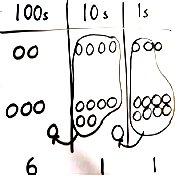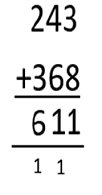###### Conceptual variation; different ways to ask children to solve 21 + 34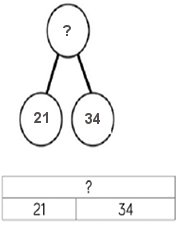Word problems:

In year 3, there are 21 children and in year 4, there are 34 children.

How many children in total?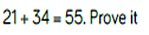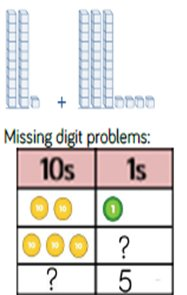### Subtraction

##### EYFS/Year 1

Taking away ones

Counting back

Find the difference

Part whole model

Making 10 using the ten frame

##### Year 2

Counting back

Find the difference

Part whole model

Make 10

Use of base 10

##### Year 3

Column method with regrouping.

(up to 3 digits using place value counters)

##### Year 4

Column method with regrouping.

(up to 4 digits)

##### Year 5

Column method with regrouping.

Abstract for whole numbers.

Start with place value counters for decimals - with the same amount of decimal places.

##### Year 6

Column method with regrouping.

Abstract methods.

Place value counters for decimals - with different amounts of decimal places.

#### Calculation Policy: Subtraction

##### Concrete

Physically taking away and removing objects from a whole (ten frames, Numicon, cubes and other items such as beanbags could be used).
4 - 3 = 1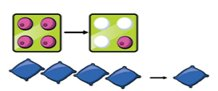##### Pictorial

Children to draw the concrete resources they are using and cross out the correct amount. The bar model can also be used.##### Abstract

4 - 3 = 7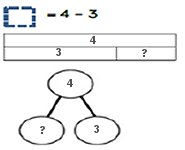Counting back (using number lines or number tracks) children start with 6 and count back 2.

6 - 2 = 4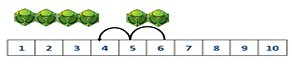Children to represent what they see pictorially e.g.Children to represent the calculation on a number line or number track and show their jumps. Encourage childen to use an empty number line.Finding the difference (using cubes, Numicon or Cuisenaire rods, other objects an calso be used).

Calculate the difference between 8 and 5.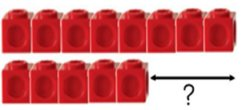Children to draw the cubes/other concrete objects which they have used or use the bar model to illustrate what they need to calculate.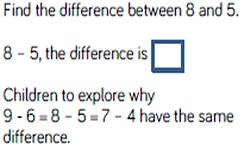Making 10 using ten frames.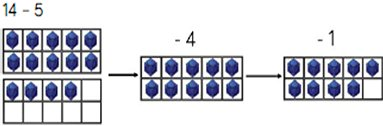Children to present the ten frame pictorially and discuss what they did to make 10.Children to show how they can make 10 by partitioning the subtrahend.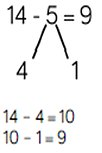Column method using base 10.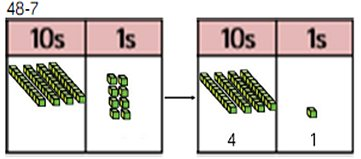Children to represent the base 10 pictorially.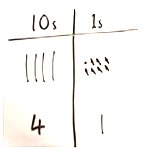Column method or children could count back 7.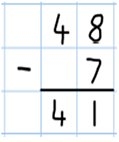Column method using base 10 and having to exchange.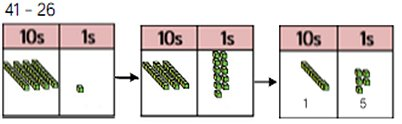Represent the base 10 pictorially, remembering to show the exchange.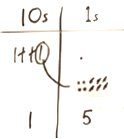Formal column method. Children must understand that when they have exchanged the 10 they still have 41 because 41 = 30 + 11.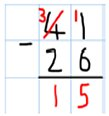Column method using place value counters.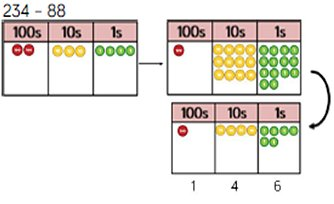Represent the place value counters pictorially; remembering to show what has been exchanged.Formal column method. Children must understand what has happened when they have crossed out the digits.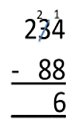###### Conceptual variation; different ways to ask children to solve 391 - 186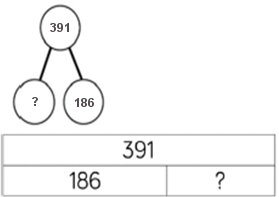Raj spent £391, Timmy spent £186.
How much more did Raj spend?

Calculate the difference between 391 and 186.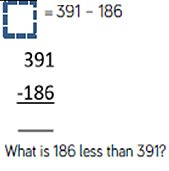Missing digit Calculations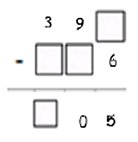### Multiplication

##### EYFS/Year 1

Recognising and making equal groups

Doubling

Counting in multiples - use cubes, Numicon and other objects in the classroom

##### Year 2

Arrays - showing commutative multiplication

##### Year 3

Arrays

2d x 1d using base 10

##### Year 4

Column multiplication - introduced with place value counters.

(2 and 3 digit multiplied by 1 digit)

##### Year 5

Column multiplication

Abstract only but might need a repeat of year 4 first (up to 4 digit numbers multiplied by 1 or 2 digits)

##### Year 6

Column multiplication

Abstract methods (multi-digit up to 4 digits by a 2 digit number)

#### Calculation Policy: Multiplication

##### Concrete

3 x 4
4 + 4 + 4
There are 3 equal groups, with 4 in each group##### Pictorial

Children to represent the practical resources in a picture and use a bar model.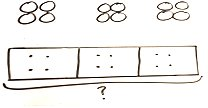##### Abstract

3 x 4 = 12
4 + 4 + 4 = 12

Number lines to show repeated groups
3 x 4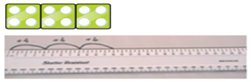Cuisenaire rods can be used too.

Represent this pictorially alongside a number ine e.g.: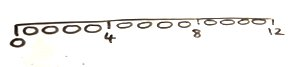Abstract number line showing three jumps of four.

3 x 4 = 12Use arrays to illustrate commutativity counters and other objects can also be used.

2 x 5 = 5 x 2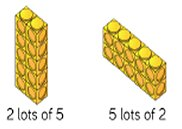Children to represent the arrays pictorially.Children to be able to use an array to write a range of calculations e.g.:

10 = 2 x 5
5 x 2 = 10
2 + 2 + 2 + 2 + 2 = 10
10 = 5 + 5

MPartition to multiply using Numicon, base 10 or Cuisenaire rods.
4 x 15Children to represent the concrete manipulatives pictorially.Children to be encouraged to show the steps they have taken.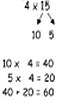A number line can also be used.Formal column method with place value counters (base 10 can also be used). 3 x 23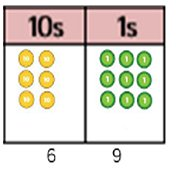Children to represent the counters pictorially.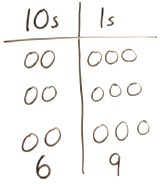Children to record what it is they are doing to show understanding.Formal column method with place value counters.
6 x 23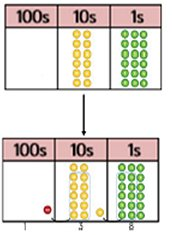Children to represent the counters/base 10, pictorially e.g. the image below.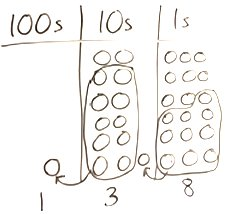Formal written method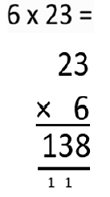When children start to multiply 3d x 3d and 4d x 2d etc, they should be confident with the abstract:

To get 744 children have solved 6 x 124.
To get 2480 they have solved 20 x 124.###### Conceptual variation; different ways to ask children to solve 6 x 23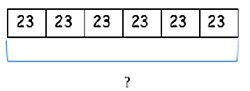Mai had to swim 23 lengths, 6 times a week.
How many lengths did she swim in one week?

With the counters, prove that 6 x 23 = 138

Find the product of 6 and 23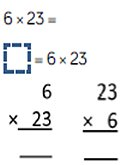What is the calculation>
What is the product?### Division

##### EYFS/Year 1

Sharing objects into groups.

Division as grouping e.g. I have 12 sweets and put them in groups of 3, how many groups?

use cubes and draw round 3 cubes at a time.

##### Year 2

Division as grouping

Division within arrays - linking to multiplication

Repeated subtraction

##### Year 3

Division with a remainder - using lollipop sticks, times tables facts and repeated subtraction.

2d divided by 1d using base 10 or place value counters

##### Year 4

Division with a remainder

Short division (up to 3 digits by 1 digit - concrete and pictorial)

##### Year 5

Short division

(up to 4 digits by a 1 digit number including remainders)

##### Year 6

Short division

Long division with place value counters (up to 4 digits by a 2 digit number)

Children should exchange into the tenths and hundredths column too

#### Calculation Policy: Division

##### Concrete

Sharing using a range of objects.
6 ÷ 2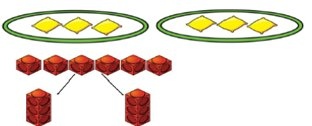##### Pictorial

Represent the sharing pictorially.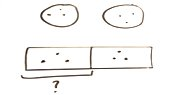##### Abstract

6 ÷ 2 = 3Children should also be encouraged to use their 2 times tables facts.

Repeated subtraction using Cuisenaire rods above a ruler.

6 ÷ 2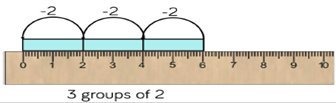Children to represent repeated subtraction pictorially.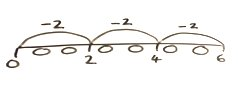Abstract number line to represent the equal groups that have been subtracted.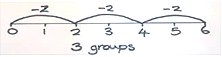2d ÷ 1d with remainders using lollipop sticks. CUisenaire rods, above a ruler can also be used.
13 ÷ 4

Use of lollipop sticks to form wholes - squares ae made because we are dividing by 4.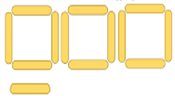There are 3 whole squares, with 1 left over.

Children to represent the lollipop sticks pictorially.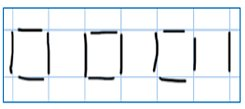There are 3 whole squares, with 1 left over.

13 ÷ 4 - 3 remainder 1

Children should be encourated to use their times table facts; they could also represent repeated addition on a number line.

'3 groups of 4, with 1 left over'Sharing using place value counters.
42 ÷ 3 = 14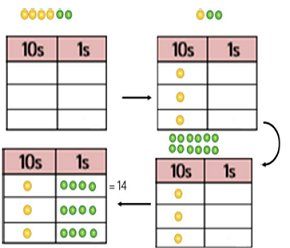Children to represent the place value counters pictorially.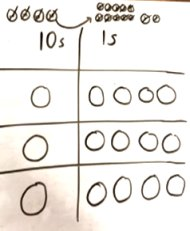Children to be able to make sense of the place value counters and write calculations to show the process.

42 ÷ 3
42 = 30 + 12
30 ÷ 3 = 10
12 ÷ 3 = 4
10 + 4 = 14

Short division using place value counters to group.
516 ÷ 5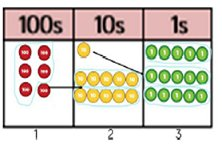1. Make 615 with place value counters.
2. How many groups of 5 hundreds can you make with 6 hundred counters?
3. Exchange 1 hundred for 10 tens.
4. How many groups of 5 tens can you make with 11 ten counters?
5. Exchange 1 ten for 10 ones..
6. How many groups of 5 ones can you make with 15 ones?

Represent the place value counters pictorially.Children to **** the calculation using the short division scaffold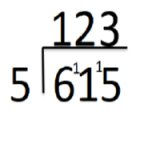Long division using place value counters.
2544 ÷ 12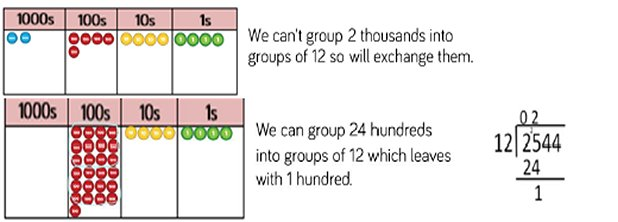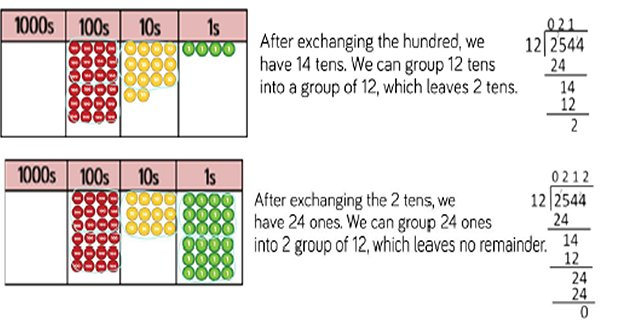###### Conceptual variation; different ways to ask children to solve 615 ÷ 5

Using the part whole model below, how can you divide 615 by 5 without using short division?I have £615 and share it equally between 5 bank accounts. How much will be in each account?

615 pupils need to be put into 5 groups. How many will be in each group?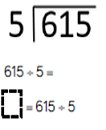What is the calculation?